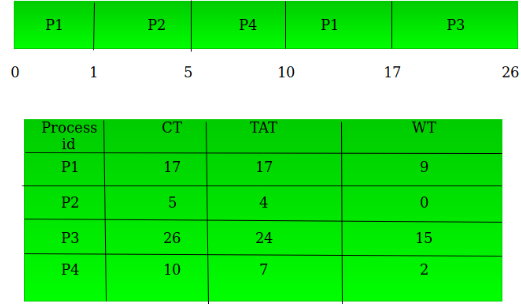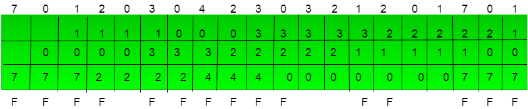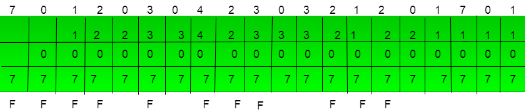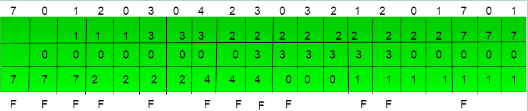# UGC NET COMPUTER SCIENCE SOLVED PAPERS 2017-19 - UGC NET Computer Science November 2017 Paper 3

>>>>>>>>UGC NET Computer Science November 2017 Paper 3

• A

(16, 3, 5)• B

(8, 5, 3)• C

(8, 3, 5)• D

(16, 4, 4)• A

10.52 months• B

11.52 months• C

12.52 months• D

14.52 months• Option : B
• Explanation :
20000 LOC = 20k LOC
Ef fort = a * (KLOC)b PM
Tdev = c * (Ef fort)d Months
Ef fort = 2.4*(20)1.05 PM = 55.756 PM
Tdev = 2.5*(55.756)0.38 Months = 11.52 Months

• A

Only (a), (c), and (d)• B

Only (a), (b), and (c)• C

Only (a), (b), and (d)• D

Only (b), (c), and (d)• Option : A
• Explanation :
Managing several versions or releases of a software is manged by SCM Filing bug reports and tracking their progressis not manged by SCM Allowing team members to work in parallel is manged by SCM Identifying when and where a regression occurred is manged by SCM So, option (A) is correct.

 Process Arrival Time Burst Time P1 0 8 P2 1 4 P3 2 9 P4 3 5
The average waiting time for preemptive SJF scheduling algorithm is __________.

• A

6.5• B

7.5• C

6.75• D

7.75• Option : A
• Explanation :
First we will make gantt chart of given process then we will calculate turn around time and waiting time of individual process.Now we have to calculate average waiting time for schedule:
avg waiting time = wt(P1 + P2 + P3 + P4 )/number of process.
ie. (9 + 0 + 15 + 2) / 4
= 26 / 4
= 6.5
So, option (A) is correct.

• A

FIFO• B

LIFO• C

LRU• D

Optimal• Option : D
• Explanation :1. In FIFO algorithm 15 page fault will occur.2. In LIFO algorithm 11 page fault will occur.3. In LRU algorithm 12 page fault will occur.4.In optimal algorithm 9 page fault will occur.
So, option (D) will be correct.